Snowflake is a well known cloud-based database. It’s used for Data Warehouses and other big data applications.

In this article and the following ones I want to show how to setup and access a snowflake database from various clients such as R, Tableau and PowerBI.

So let’s start using R.

## Setup Snowflake

Assuming that we already have access to an instance of Snowflake we first setup a new environment for our tests. This environment consists of a warehouse, a database and an user.

Note: I will name all things we will create EXAMPLE_something. So we can recognize our new items.

### Create Warehouse

A warehouse represents the computing power. A warehouse has a specific size which determines the computing power (and the price you pay for it). You only pay for the time the warehouse is used.

So switch to SYSADMIN role and create a new warehouse. We call it EXAMPLE_WH.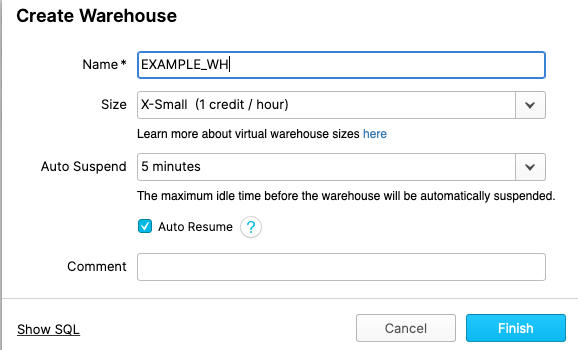Don’t press Finish. Instead click onto Show SQL.

As you can see we can use SQL-commands (or SQL-like commands) to create this warehouse. Actually we’ll do this.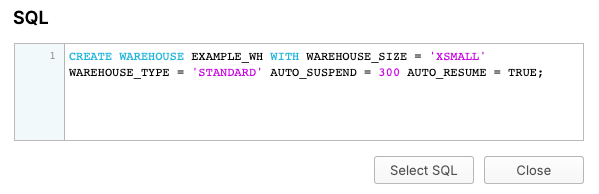Copy this SQL-command and switch to Worksheets. As you can see we choose a smaller timeout for AUTO_SUSPEND. We also lower the value for STATEMENT_TIMEOUT_IN_SECONDS. That’s like a safety net in case something runs amok.

 ``````1 2 3 4 5 `````` ``````CREATE WAREHOUSE EXAMPLE_WH WITH WAREHOUSE_SIZE = 'XSMALL' WAREHOUSE_TYPE = 'STANDARD' AUTO_SUSPEND = 60 AUTO_RESUME = TRUE; alter warehouse "EXAMPLE_WH" set STATEMENT_TIMEOUT_IN_SECONDS = 60; show parameters in warehouse "EXAMPLE_WH"; ``````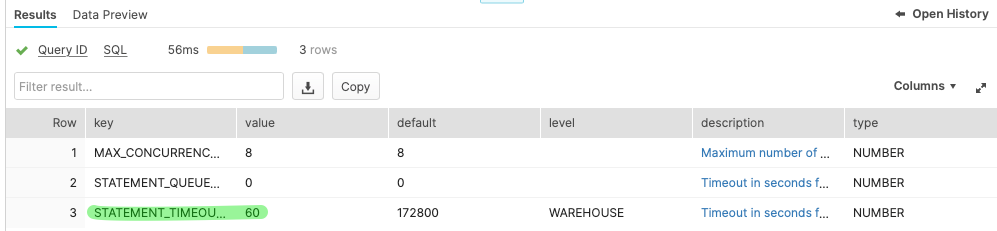### Create Database

Using the worksheet we can create a database, too. We call it EXAMPLE_DB.

 ``````1 `````` ``````CREATE DATABASE EXAMPLE_DB; ``````

### Create Role

We also create a role called EXAMPLE_ROLE. This role will be used to manage permissions.

 ``````1 2 `````` ``````use role USERADMIN; CREATE ROLE EXAMPLE_ROLE; ``````

### Create User

Now we can switch our own role to ACCOUNTADMIN and create a new user called EXAMPLE_USER.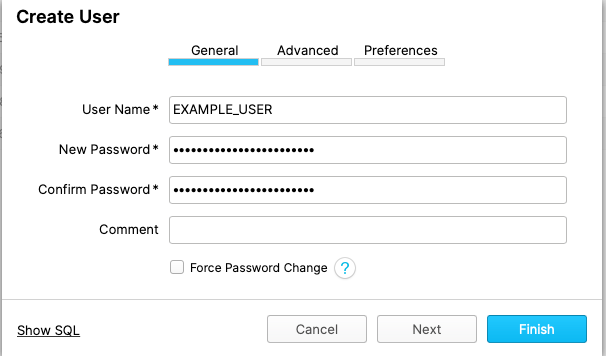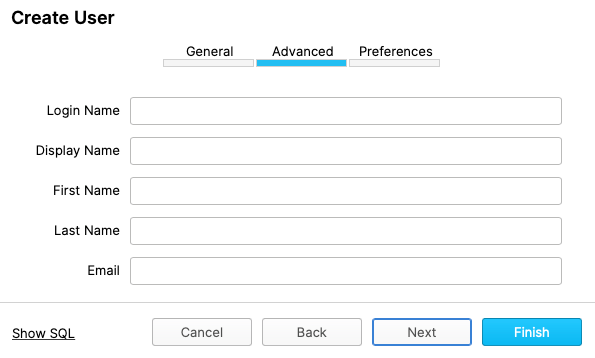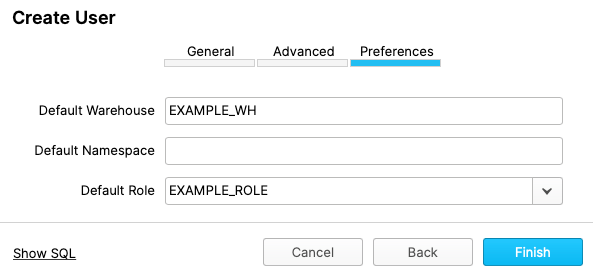As you can see it’s also possible to create a user using SQL-commands.

 ``````1 2 `````` ``````CREATE USER EXAMPLE_USER PASSWORD = '****' DEFAULT_ROLE = "EXAMPLE_ROLE" DEFAULT_WAREHOUSE = 'EXAMPLE_WH' MUST_CHANGE_PASSWORD = FALSE; GRANT ROLE "EXAMPLE_ROLE" TO USER EXAMPLE_USER; ``````

### Grant permissions to Role

Now grant permissions for the database to our new role.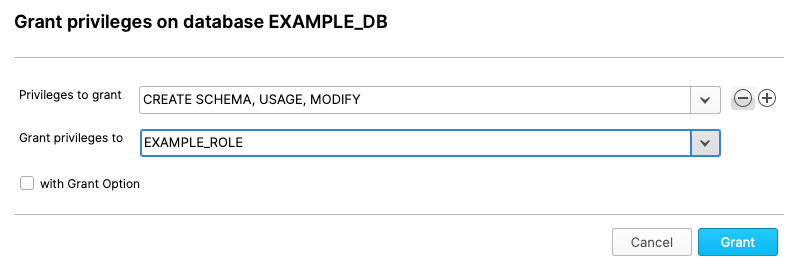Also grant permissions for the warehouse to the role.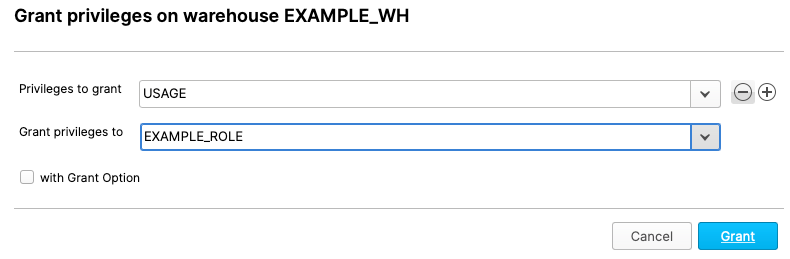### Create Schema

Now we should grant EXAMPLE_ROLE to SYSADMIN role because we can use our default user and switch to EXAMPLE_ROLE and create a schema within the database.

The new schema is called EXAMPLE_SCHEMA.

 ``````1 2 3 `````` ``````use role EXAMPLE_ROLE; use database EXAMPLE_DB; CREATE SCHEMA "EXAMPLE_DB"."EXAMPLE_SCHEMA"; ``````

### Create tables

Within the newly created schema we create two empty tables.

 ``````1 `````` ``````CREATE TABLE "EXAMPLE_DB"."EXAMPLE_SCHEMA"."TABLE_A" ("ID_1" INTEGER NOT NULL, "ID_2" STRING NOT NULL, "VALUE_1" STRING NOT NULL, "VALUE_2" INTEGER NOT NULL); ``````
 ``````1 `````` ``````CREATE TABLE "EXAMPLE_DB"."EXAMPLE_SCHEMA"."TABLE_B" ("ID_1" INTEGER NOT NULL, "ID_2" STRING NOT NULL, "VALUE_1" STRING NOT NULL, "VALUE_2" INTEGER NOT NULL); ``````

## Accessing Snowflake from R

So now it’s time to connect to Snowflake from R:

### Connection via DBI

You have to setup an odbc-connection to access Snowflake from R. The way to do this depends on your operation system. So have a look at the manual at Snowflake, please.

 `````` 1 2 3 4 5 6 7 8 9 10 11 12 13 `````` ``````library(tidyverse) library(dbplyr) library(DBI) db_connection <- DBI::dbConnect(odbc::odbc(), Driver="Snowflake", Server="MY_SNOWFLAKE_SERVER.snowflakecomputing.com", Database="EXAMPLE_DB", SCHEMA="EXAMPLE_SCHEMA", UID="EXAMPLE_USER", PWD="MY_SECRET_PASSWORD", WAREHOUSE="EXAMPLE_WH" ) ``````

### Writing some data

Let’s generate some sample data and push it to Snowflake.

 `````` 1 2 3 4 5 6 7 8 9 10 11 12 13 14 15 16 17 18 19 20 `````` ``````n_rows <- 12 set.seed(0815) data_a <- data.frame( ID_1 = 1:n_rows, ID_2 = c("a", "b", "c"), VALUE_1 = sample(c("Apple", "Pear", "Banana"), n_rows, replace = TRUE), VALUE_2 = round(runif(n_rows, max = 100)) ) copy_to(db_connection, data_a, "TABLE_A", overwrite = TRUE) ids <- 12 n_rows <- ids * 10 set.seed(0815) data_b <- data.frame( ID_1 = 1:ids, ID_2 = c("a", "b", "c"), VALUE_1 = sample(c("Jim", "Max", "Hanna", "Maria", "Lucy", "John"), n_rows, replace = TRUE), VALUE_2 = round(runif(n_rows, max = 100)) ) copy_to(db_connection, data_b, "TABLE_B", overwrite = TRUE) ``````

As you can see we use `copy_to()` to push a data.frame into a Snowflake database. That’s not the preferred way. For small data it’s acceptable and a fast way to get some data to Snowflake.

Reading from Snowflake is as simple as working with data.frames. We just bind a table to an R object using `tbl()`.

 ``````1 2 3 `````` ``````a <- tbl(db_connection, "TABLE_A") a %>% collect() ``````
 `````` 1 2 3 4 5 6 7 8 9 10 11 12 13 14 15 `````` ``````# A tibble: 12 × 4 ID_1 ID_2 VALUE_1 VALUE_2 1 1 a Pear 64 2 12 c Pear 67 3 11 b Banana 91 4 10 a Banana 4 5 9 c Pear 65 6 8 b Pear 67 7 7 a Banana 20 8 6 c Pear 33 9 5 b Banana 32 10 4 a Pear 33 11 3 c Pear 43 12 2 b Apple 91 ``````

#### Complex statements

Even complext statements you use within `tidyverse` are possible.

 ``````1 2 3 4 5 6 7 `````` ``````a %>% select(ID_1, ID_2, VALUE_1) %>% left_join(b, by = c("ID_1" = "ID_1", "ID_2" = "ID_2")) %>% group_by(ID_1, ID_2, VALUE_1.x, VALUE_1.y) %>% summarize(VALUE_2 = sum(VALUE_2)) %>% arrange(ID_1) %>% collect() ``````
 `````` 1 2 3 4 5 6 7 8 9 10 11 12 13 `````` ``````# A tibble: 59 × 5 # Groups: ID_1, ID_2, VALUE_1.x  ID_1 ID_2 VALUE_1.x VALUE_1.y VALUE_2 1 1 a Pear John 58 2 1 a Pear Hanna 174 3 1 a Pear Jim 85 4 1 a Pear Max 30 5 1 a Pear Maria 171 6 2 b Apple Hanna 261 7 2 b Apple John 116 8 2 b Apple Lucy 162 9 2 b Apple Jim 170 ``````

The last command `collect()` fetches the data from the database. Until this command nothing has happend.

#### SQL

Before calling `collect()` we can inspect the SQL-code which will be send to Snowflake.

Therefor you can call `show_query()`.

 ``````1 2 3 4 5 6 7 8 `````` ``````query <- a %>% select(ID_1, ID_2, VALUE_1) %>% left_join(b, by = c("ID_1" = "ID_1", "ID_2" = "ID_2")) %>% group_by(ID_1, ID_2, VALUE_1.x, VALUE_1.y) %>% summarize(VALUE_2 = sum(VALUE_2)) %>% arrange(ID_1) query %>% show_query() ``````
 `````` 1 2 3 4 5 6 7 8 9 10 `````` `````` SELECT "ID_1", "ID_2", "VALUE_1.x", "VALUE_1.y", SUM("VALUE_2") AS "VALUE_2" FROM (SELECT "LHS"."ID_1" AS "ID_1", "LHS"."ID_2" AS "ID_2", "LHS"."VALUE_1" AS "VALUE_1.x", "RHS"."VALUE_1" AS "VALUE_1.y", "VALUE_2" FROM (SELECT "ID_1", "ID_2", "VALUE_1" FROM "TABLE_A") "LHS" LEFT JOIN "TABLE_B" AS "RHS" ON ("LHS"."ID_1" = "RHS"."ID_1" AND "LHS"."ID_2" = "RHS"."ID_2") ) "q01" GROUP BY "ID_1", "ID_2", "VALUE_1.x", "VALUE_1.y" ORDER BY "ID_1" ``````

Here’s the SQL-command which Snowflake shows in its history: It’s identical.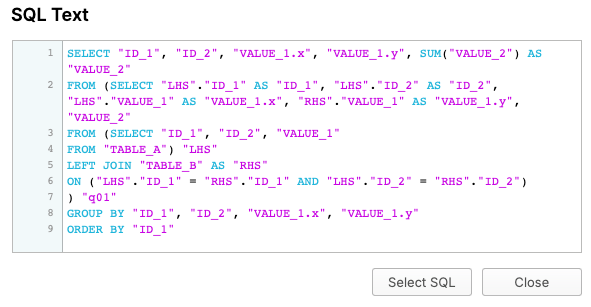## Next Articles

The next articles will show how to access this data from Tableau and PowerBI.